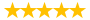# gb550 unit 5 quiz

Question # 00004521 Posted By: neil2103 Updated on: 12/03/2013 05:28 PM Due on: 12/31/2013
Subject Finance Topic Finance Tutorials:
Question
1. Teall Development Company hired you as a consultant to help them estimate its cost of capital. You have been provided with the following data: D1 = \$1.45; P0 = \$22.50; and g = 6.50% (constant). Based on the DCF approach, what is the cost of common from retained earnings? (Points : 2)

 Question 2. 2. Assume that you are a consultant to Broske Inc., and you have been provided with the following data: D1 = \$0.67; P0 = \$27.50; and g = 8.00% (constant). What is the cost of common from retained earnings based on the DCF approach? (Points : 2)

 Question 3. 3. Anderson Systems is considering a project that has the following cash flow and WACC data. What is the project's NPV? Note that if a project's expected NPV is negative, it should be rejected. WACC: 9.00%Year 0 1 2 3 Cash flows -\$1,000 \$500 \$500 \$500 (Points : 2)

 Question 4. 4. A company’s perpetual preferred stock currently sells for \$92.50 per share, and it pays an \$8.00 annual dividend. If the company were to sell a new preferred issue, it would incur a flotation cost of 5.00% of the issue price. What is the firm's cost of preferred stock? (Points : 2)

 Question 5. 5. DeLong Inc. has fixed operating costs of \$470,000, variable costs of \$2.80 per unit produced, and its products sell for \$4.00 per unit. What is the company's breakeven point, i.e., at what unit sales volume would income equal costs? (Points : 2)

 Question 6. 6. Assume a project has normal cash flows. All else equal, which of the following statements is CORRECT? (Points : 2)

 Question 7. 7. For capital budgeting and cost of capital purposes, the firm should assume that each dollar of capital is obtained in accordance with its target capital structure, which for many firms means partly as debt, partly as preferred stock, and partly common equity. (Points : 2)

 Question 8. 8. Which of the following statements is CORRECT? (Points : 2)

 Question 9. 9. The cost of equity raised by retaining earnings can be less than, equal to, or greater than the cost of external equity raised by selling new issues of common stock, depending on tax rates, flotation costs, the attitude of investors, and other factors. (Points : 2)

 Question 10. 10. Which of the following statements is CORRECT? (Points : 2)

Tutorials for this Question
1. ## Solution: gb550 unit 5 quiz

Tutorial # 00004318 Posted By: neil2103 Posted on: 12/03/2013 05:51 PM
Puchased By: 3
Tutorial Preview
The solution of gb550 unit 5 quiz...
Attachments
Gb550_quiz_5.docx (49.41 KB)
gb550_unit5_5.docx (14.75 KB)
Recent Feedback
Rated By Feedback Comments Rated On
kc...804Quality assignments at affordable prices 07/15/2015
2. ## Solution: GB550 Unit 1 quiz

Tutorial # 00006231 Posted By: duckhead20 Posted on: 01/13/2014 10:48 PM
Puchased By: 2
Tutorial Preview
compounding? (Points : 2) \$622.20 \$689.42 \$1,928.78 \$2,131.81 Question 4. 4. Suppose a ...
Attachments
3. ## Solution: GB550 Unit 2 quiz

Tutorial # 00006232 Posted By: duckhead20 Posted on: 01/13/2014 10:57 PM
Puchased By: 2
Tutorial Preview
4.72 5.23 Question 3. 3. Lindley Corp.'s stock ...
Attachments
4. ## Solution: GB550 Unit 3 quiz

Tutorial # 00006233 Posted By: duckhead20 Posted on: 01/13/2014 11:03 PM
Puchased By: 2
Tutorial Preview
of the following events would make it more likely that ...
Attachments
Unit_3_Quiz.docx (115.18 KB)
5. ## Solution: gb550 unit 5 quiz

Tutorial # 00048923 Posted By: spqr Posted on: 03/02/2015 07:30 PM
Puchased By: 3
Tutorial Preview
The solution of gb550 unit 5 quiz...
Attachments
Solution-00048923.zip (94 KB)
Recent Feedback
Rated By Feedback Comments Rated On
Kar...liamsElaborated tutorials; all points covered 04/02/2015

Great! We have found the solution of this question!